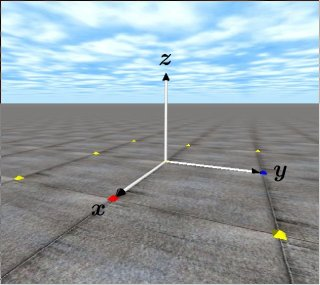# 9. Position and OrientationODE coordinate system (right handed)

This is the 9th ODE (Open Dynamics Engine) tutorial.

This time, firstly,  I explain a unit system and a coordinate of ODE. Secondly, I explain how to set and get a position and a rotation of a body.

Unit system

ODE does not mind any unit system. An angle, however, is radian. In this tutorial, the SI unit system is used. This is very familiar to us (not U.S.A). Length is [m],  weight is [kg] and time is [s].

Coordinate system

The coordinate system of ODE as shown in the above figure, is the Cartesian coordinate system and right-handed. It is widely used in physics and mathematics.

There are nine small pyramids as shown in the above figure.  The origin of the coordinate system is  the center of pyramids, the x-axis is the direction from the center to the red pyramid. The y-axis is the direction from the center to the blue pyramid. The z-axis is the direction from the center  to the sky.  These tutorials adopt the SI unit system, so the distance between pyramids is 1[m].

Setting position and posture

A position and an orientation of a body must be set.  The following APIs are used for the purpose.

• void dBodySetPosition (dBodyID, dReal x, dReal y, dReal z);
Set a position (x, y, z) in the absolute coordinate system.
• void dRFromAxisAndAngle (dMatrix3 R, dReal ax, dReal ay, dReal az, dReal angle);
Calculate a rotation matrix from a rotation axis vector (ax, ay, az) and rotational angle [rad] which rotates counterclockwisely.
• void dBodySetRotation (dBodyID, const dMatrix3 R);
Set an orientation of the body with the rotation matrix R.

In addition,  Quaternion can be used in ODE. The API is dBodySetQuaternion (dBodyID, const dQuaternion q).

Getting the position and posture

• const dReal *dBodyGetPosition (dBodyID);
Get a positon of the body. The return value is a pointer to an array.
• const dReal *dBodyGetRotation (dBodyID);
Get a ratation matrix of the body.The return value is a pointer to an array.

Sample Code
In the following sample code, a cylinder is located at (0.0, 0.0, 1.0),  and the orientation is set that the cylinder is rotated 45 degrees (M_PI/4.0) along the x-axis.

In addition, the center of gravity position of a body is displayed by printPos().

```typedef struct {
dBodyID body;
dGeomID geom;
} MyObject;

MyObject pillar;

void createPillar()
{
dMass m1;
dReal radius = 0.1, length = 0.5, mass = 1.0; // radius, length, mass

pillar.body = dBodyCreate(world);
dMassSetZero(&m1);
dBodySetMass(pillar.body,&m1);
dBodySetPosition(pillar.body,0.0,0.0,1.0); // Set a position to (0.0,0.0,1.0)[m]

dMatrix3 R;
dRFromAxisAndAngle(R,1.0,0.0,0.0, M_PI/4.0);　// Rotate PI/4[rad] along X axis
dBodySetRotation(pillar.body,R);
dGeomSetBody(pillar.geom,pillar.body);
}

void printPos(dBodyID obj_body) // print a position
{
const dReal *pos;　　// Do not forget const to prevent an error
dReal x, y, z;
pos = dBodyGetPosition(obj_body);　// Return value is an pointer to a structure
x = pos;　   y = pos;   z = pos;
printf("x=%f y=%f z=%f \n", x, y, z);
}```

That’s all.  See you !

demu

スポンサーリンク
demura.netをフォローするdemura.net

### Folding@home Kanazawa (ID 257261)

みんなのためにおうちで新型コロナウイルスを解析しよう！

タイトルとURLをコピーしました